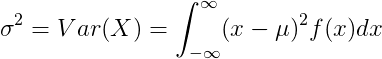# Variance

In probability theory and statistics, variance is the expectation of the squared deviation of a random variable from its mean.

### Variance definition

The variance of random variable X is the expected value of squares of difference of X and the expected value μ.

σ2 = Var(X) = E[(X – μ)2]

From the definition of the variance we can get

σ2 = Var(X) = E(X 2) – μ2

### Variance of continuous random variable

For continuous random variable with mean value μ and probability density function f(x):or### Variance of discrete random variable

For discrete random variable X with mean value μ and probability mass function P(x):or